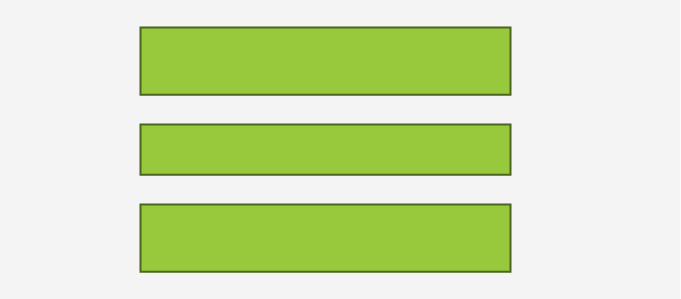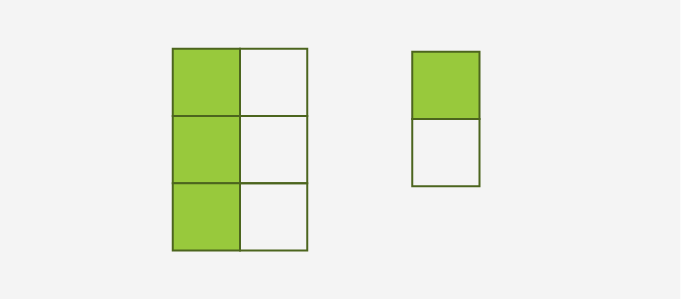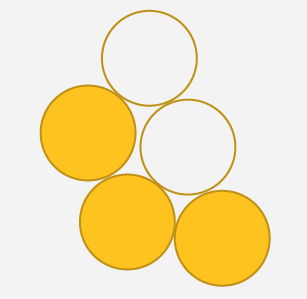# Equivalent - Definition with Examples

The Complete K-5 Math Learning Program Built for Your Child

• 40 Million Kids

Loved by kids and parent worldwide

• 50,000 Schools

Trusted by teachers across schools

• Comprehensive Curriculum

Aligned to Common Core

## Equivalent

The term “equivalent” in math refers to two values, numbers or quantities which are the same.The equivalence of two such quantities is denoted by a bar over an equal sign. It also implies logical equivalence between two values or set of quantities.## Equivalent expressions

Two mathematical expressions are said to be equivalent if they yield the same result upon solving them.

For example, let’s solve the following numerical expressions:

25 × 5 = 125

Also, 102 + 52  = 100 + 25 =125

Thus, the above two expressions are equivalent and can be written as:   25 × 5 = 102 + 52

Similarly, following two math expressions are also equivalent:

2 × (10 – 8) = 42 ÷ 12

⇒ 4

## Equivalent fractions

Two fractions are equivalent if the value, proportion, or quantity they represent is the same. Equivalent fractions can have different numerators and denominators.

For example, 3 6 = 36 72

⇒  36 72 = 12 ÷ 3 12 ÷ 6

⇒  3 6 or 1 2The figure here proportionally represents  1 2 portion of a given whole.

The numerical equivalence for the picture below is: 3 5

The  numerator denotes the colored balls and denominator denotes the total number of balls

⇒ 60%

⇒ 0.6Application

The use of the equivalent symbol (as three bars) is frequently used in Unicode programming for computers, as well as in Boolean algebra. Venn diagrams use the concept of logical equivalence to establish the relationship between two algebraic expressions and functions. The concept of equivalence is also used in explaining the structural similarity between the chemical compounds.

 Fun Facts The sign “equals” (=) was invented by a Welsh mathematician Robert Recorde in 1557. Equivalent sign and equivalence of Boolean functions were explained by 19th century mathematician George Boole.

Won Numerous Awards & Honors